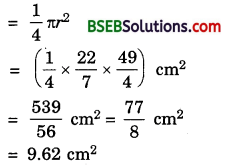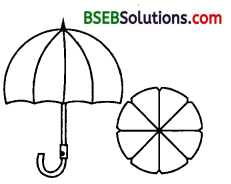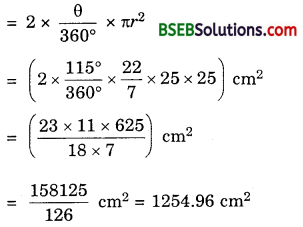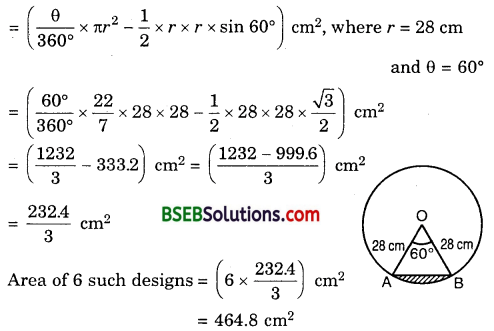# HSSlive: Plus One & Plus Two Notes & Solutions for Kerala State Board

## BSEB Class 10 Maths Chapter 12 Areas Related to Circles Ex 12.2 Textbook Solutions PDF: Download Bihar Board STD 10th Maths Chapter 12 Areas Related to Circles Ex 12.2 Book AnswersBSEB Class 10 Maths Chapter 12 Areas Related to Circles Ex 12.2 Textbook Solutions PDF: Download Bihar Board STD 10th Maths Chapter 12 Areas Related to Circles Ex 12.2 Book Answers

BSEB Class 10th Maths Chapter 12 Areas Related to Circles Ex 12.2 Textbooks Solutions and answers for students are now available in pdf format. Bihar Board Class 10th Maths Chapter 12 Areas Related to Circles Ex 12.2 Book answers and solutions are one of the most important study materials for any student. The Bihar Board Class 10th Maths Chapter 12 Areas Related to Circles Ex 12.2 books are published by the Bihar Board Publishers. These Bihar Board Class 10th Maths Chapter 12 Areas Related to Circles Ex 12.2 textbooks are prepared by a group of expert faculty members. Students can download these BSEB STD 10th Maths Chapter 12 Areas Related to Circles Ex 12.2 book solutions pdf online from this page.

## Bihar Board Class 10th Maths Chapter 12 Areas Related to Circles Ex 12.2 Books Solutions

 Board BSEB Materials Textbook Solutions/Guide Format DOC/PDF Class 10th Subject Maths Chapter 12 Areas Related to Circles Ex 12.2 Chapters All Provider Hsslive

## How to download Bihar Board Class 10th Maths Chapter 12 Areas Related to Circles Ex 12.2 Textbook Solutions Answers PDF Online?

2. Click on the Bihar Board Class 10th Maths Chapter 12 Areas Related to Circles Ex 12.2 Answers.
3. Look for your Bihar Board STD 10th Maths Chapter 12 Areas Related to Circles Ex 12.2 Textbooks PDF.
4. Now download or read the Bihar Board Class 10th Maths Chapter 12 Areas Related to Circles Ex 12.2 Textbook Solutions for PDF Free.

## BSEB Class 10th Maths Chapter 12 Areas Related to Circles Ex 12.2 Textbooks Solutions with Answer PDF Download

Find below the list of all BSEB Class 10th Maths Chapter 12 Areas Related to Circles Ex 12.2 Textbook Solutions for PDF’s for you to download and prepare for the upcoming exams:

## BSEB Bihar Board Class 10th Maths Solutions Chapter 12 Areas Related to Circles Ex 12.2

Question 1.
Find the area of a sector of a circle with radius 6 cm, if angle of the sector is 60°.
Solution:
We know that the area A of a sector of angle θ in a circle of radius r is given by A = 𝜃360∘ x πr².
Here, r = 6 cm, θ = 60°
∴ A = [60∘360∘×227×36] cm² = (1327) cm²
= 18.86 cm²

Question 2.
Find the area of a quadrant of a circle whose circumference is 22 cm.
Solution:
Let r cm be the radius of the circle. Then,
Circumference = 22 cm means 2πr = 22
So, 2 x 227 x r = 22
or r = 72
So, radius of the circle is 72 cm.
Area of the quadrant of a circleQuestion 3.
The length of the minute hand of a clock is 14 cm. Find the area swept by the minute hand in 5 minutes.
Solution:
Clearly, minute hand of a clock describes a circle of radius equal to its length, i.e., r = 14 cm.
Since the minute hand rotates through 6° in one minute, therefore area swept by the minute hand in one minute is the area of a sector of angle 6° in a circle of radius 14 cm.
Hence, the required area i.e., the area swept in 5 minutesQuestion 4.
A chord of a circle of radius 10 cm subtends a right angle at the centre. Find the area of the corresponding : (i) minor segment (ii) major sector (Use n = 3.14)
Solution:
Here, r = 10 cm and θ = 90°.
(i) Area of the minor segmentAliter. Area of the minor segment = Area of the sector – Area of right triangle(ii) Area of the major sectorQuestion 5.
In a circle of a radius 21 cm, an arc subtends an angle of 60° at the centre. Find :
(i) the length of the arc
(ii) area of the sector formed by the arc
(iii) area of the segment formed by the corresponding chord.
Solution:
Here, r = 21 cm and θ = 60°
(i) Length of the arc, l
= 𝜃180∘ x πr
= (60∘180∘×227×21) cm = 22 cm
(ii) Area of the sector, A
= 𝜃360∘ x πr²
= (60∘360∘×227×21×21) cm² = 231 cm²
(iii) Area of the segmentQuestion 6.
A chord of a circle of radius 15 cm subtends an angle of 60° at the centre. Find the areas of the corresponding minor and major segments of the circle. (Use π = 3.14 and 3‾√ = 1.73).
Solution:
Here, r = 15 cm and θ = 60°.
∴ Area of the minor segmentArea of the major segment = Area of the circle – Area of the minor segment
= (3.14 x 225 – 20.4375)cm²
= (706.5 – 20.4375)cm²
= 686.0625 cm²

Question 7.
A chord of a circle of radius 12 cm subtends an angle of 120° at the centre. Find the area of the corresponding segment of the circle.
(Use π = 3.14 and 3‾√ = 1.73)
Solution:
Here, r = 12 cm and 0 = 120°Area of the corresponding segment of the circle = Area of the minor segment (Shown shaded in the figure)
= Area of sector OAB – Area of ∆ OAB … (1)
Now, area of sector OAB
= 120∘360∘ x 3.14 x 12² cm² = 3.14 x 48 cm² … (2)
For area of ∆ OAB, draw OM ⊥ AB. So, AM = BM and ∠AOM = ∠BOM = 60°
Now, 𝑂𝑀𝑂𝐴 = cos 60°.
Therefore, OM = OA cos 60°
= 12 x 12 cm = 6 cm.
Also, 𝐴𝑀𝑂𝐴 = sin 60°.
So, AM = OA sin 60° = 12 x 3√2 cm = 63‾√ cm
∴ AB = 2 x 63‾√ cm = 123‾√ cm
Hence, area of ∆ OAB
= 12AB x OM
= 12 x 123‾√ x 6 cm² = 363‾√ cm² … (3)
So, from (1), (2) and (3), area of the minor segment
= (3.14 x 48 – 363‾√) cm²
= (3.14 x 48 -36 x 1.73) cm²
= 12(12.56 – 3 x 1.73) cm²
= 12(12.56 – 5.19) cm²
= 12 x 7.37 cm²
= 88.44 cm²

Question 8.
A horse is tied to a peg at one corner of a square shaped grass field of side 15 m by means of a 5 m long rope (see figure). Find(i) the area of that part of the field in which the horse can graze.
(ii) the increase in the grazing area if the rope were 10 m long instead of 5 m. (Use π = 3.14)
Solution:
(i) The horse will graze over a quadrant of a circle with centre at the corner A of the field and radius AF = 5 m.
Then, the area of the quadrant of this circle= 𝜋×524 m² = 3.14×254 m²
= 78.54m² = 19.625 m²

(ii) In the 2nd case, radius = 10 m.
The area of the quadrant of this circle= 𝜋×1024 m² = 3.14×1004 m²
= 3144m² = 78.5 m²
∴ Increase in the grazing area = (78.5 – 19.625) m² = 58.875 m²

Question 9.
A brooch is made with silver wire in the form of a circle with diameter 35 mm. The wire is also used in making 5 diameters which divide the circle into 10 equal sectors as shown in the figure.Find :
(i) the total length of the silver wire required.
(ii) the area of each sector of the brooch.
Solution:
(i) Silver wire used to make a brooch
= 2πr, where r = 352 mm
= 2 x 227 x 352 mm = 110 mm
Wire used in 5 diameters = 5 x 35 mm = 175 mm
∴ Total wire used = (110 + 175) mm = 285 mm.

(ii) The area of each sector of the broochQuestion 10.
An umbrella has 8 ribs which are equally spaced (see figure). Assuming umbrella to be a flat circle of radius 45 cm, find the area between the two consecutive ribs of the umbrella.Solution:
Area between two consecutive ribs
= 18 x πr², where r = 45 cm
= (18 x 227 x 45 x 45 ) cm²
= 2227528 cm² = 795.53 cm²

Question 11.
A car has two wipers which do not overlap. Each wiper has a blade of length 25 cm sweeping through an angle of 115°. Find the total area cleaned at each sweep of the blades.
Solution:
Here, r = 25 cm and θ = 115°.
Total area cleaned at each sweep of the blades = 2 x Area of the sector
(having r = 25 cm and θ = 115°)Question 12.
To warn ships for underwater rocks, a lighthouse spreads a red coloured light over a sector of angle 80° to a distance of 16.5 km. Find the area of the sea over which the ships are warned. (Use π = 3.14)
Solution:
Area of the sea over which the ships are warnedQuestion 13.
A round table cover has six equal designs as shown in figure. If the radius of the cover is 28 cm, find the cost of making the designs at the rate of Rs 0.35 per cm².(Use 3‾√ = 1.7)
Solution:
Clearly from the figure,
Area of one design
= Area of the sector AOB – Area of ∆ AOBCost of making such designs at the rate of Rs 0.35 per cm²
= Rs (0.35 x 464.8)
= Rs 162.68

Question 14.
Tick the correct answer in the following :
Area of a sector of angle p (in degrees) of a circle with radius R is
(A) 𝑝180 x 2πR
(B) 𝑝180 x πR²
(C) 𝑝360 x 2πR
(D) 𝑝720 x 2πR²
Solution:
We know that area A of a sector of angle 0 in a circle of radius r is given by
A = 𝜃360∘ x πr²
But here, r = R and θ = p°.
∴ A = 𝑝∘360∘ x πR² = 𝑝720 x 2πR²
∴ (D) is the correct answer.

## Bihar Board Class 10th Maths Chapter 12 Areas Related to Circles Ex 12.2 Textbooks for Exam Preparations

Bihar Board Class 10th Maths Chapter 12 Areas Related to Circles Ex 12.2 Textbook Solutions can be of great help in your Bihar Board Class 10th Maths Chapter 12 Areas Related to Circles Ex 12.2 exam preparation. The BSEB STD 10th Maths Chapter 12 Areas Related to Circles Ex 12.2 Textbooks study material, used with the English medium textbooks, can help you complete the entire Class 10th Maths Chapter 12 Areas Related to Circles Ex 12.2 Books State Board syllabus with maximum efficiency.

## FAQs Regarding Bihar Board Class 10th Maths Chapter 12 Areas Related to Circles Ex 12.2 Textbook Solutions

#### How to get BSEB Class 10th Maths Chapter 12 Areas Related to Circles Ex 12.2 Textbook Answers??

Students can download the Bihar Board Class 10 Maths Chapter 12 Areas Related to Circles Ex 12.2 Answers PDF from the links provided above.

#### Can we get a Bihar Board Book PDF for all Classes?

Yes you can get Bihar Board Text Book PDF for all classes using the links provided in the above article.

## Important Terms

Bihar Board Class 10th Maths Chapter 12 Areas Related to Circles Ex 12.2, BSEB Class 10th Maths Chapter 12 Areas Related to Circles Ex 12.2 Textbooks, Bihar Board Class 10th Maths Chapter 12 Areas Related to Circles Ex 12.2, Bihar Board Class 10th Maths Chapter 12 Areas Related to Circles Ex 12.2 Textbook solutions, BSEB Class 10th Maths Chapter 12 Areas Related to Circles Ex 12.2 Textbooks Solutions, Bihar Board STD 10th Maths Chapter 12 Areas Related to Circles Ex 12.2, BSEB STD 10th Maths Chapter 12 Areas Related to Circles Ex 12.2 Textbooks, Bihar Board STD 10th Maths Chapter 12 Areas Related to Circles Ex 12.2, Bihar Board STD 10th Maths Chapter 12 Areas Related to Circles Ex 12.2 Textbook solutions, BSEB STD 10th Maths Chapter 12 Areas Related to Circles Ex 12.2 Textbooks Solutions,
Share: